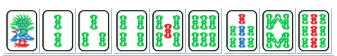？？？puts("nan")

## A

1. 一个 $2$ 的木棍和一个 $3$ 的木棍绑成一个 $5$ 的木棍，能搞多少搞多少。
2. 一个 $2$ 的木棍和两个 $1$ 的木棍绑成一个 $5$ 的木棍，能搞多少搞多少。
3. 两个 $2$ 的木棍和一个 $1$ 的木棍绑成一个 $5$ 的木棍，能搞多少搞多少。
4. 一个 $2$ 的木棍和三个 $1$ 的木棍绑成一个 $5$ 的木棍，能搞多少搞多少。
5. 五个 $1$ 的木棍绑成一个 $5$ 的木棍，能搞多少搞多少。

sol A

sol B

sol C

## D

Pure Straight 指一气通贯，就是拥有同种数牌的 123 456 789 三个顺子。门前两番，副露（鸣牌）一番，下图例子即与清一色复合，还多见于与混一色复合。容易平和。$K$ 的范围很小，我们可以用状压来表示 $f_{S}$ 为当前选的数集合为 $S$，搞出这个排列的最小次数。加入我们选出的是 $I=(i_1,i_2,\dots,i_K)$ ，我们要把它排成一个有序的 $J=(j_1, j_2,\dots,j_K)$ 。要最小这个操作步数。结论太强了：记 $m = \lceil \frac{K}{2} \rceil$，那么 $i_m = j_m$。明确一件事情：当 $I$ 确定的时候， $J$ 就被确定了。

sol D

sol E